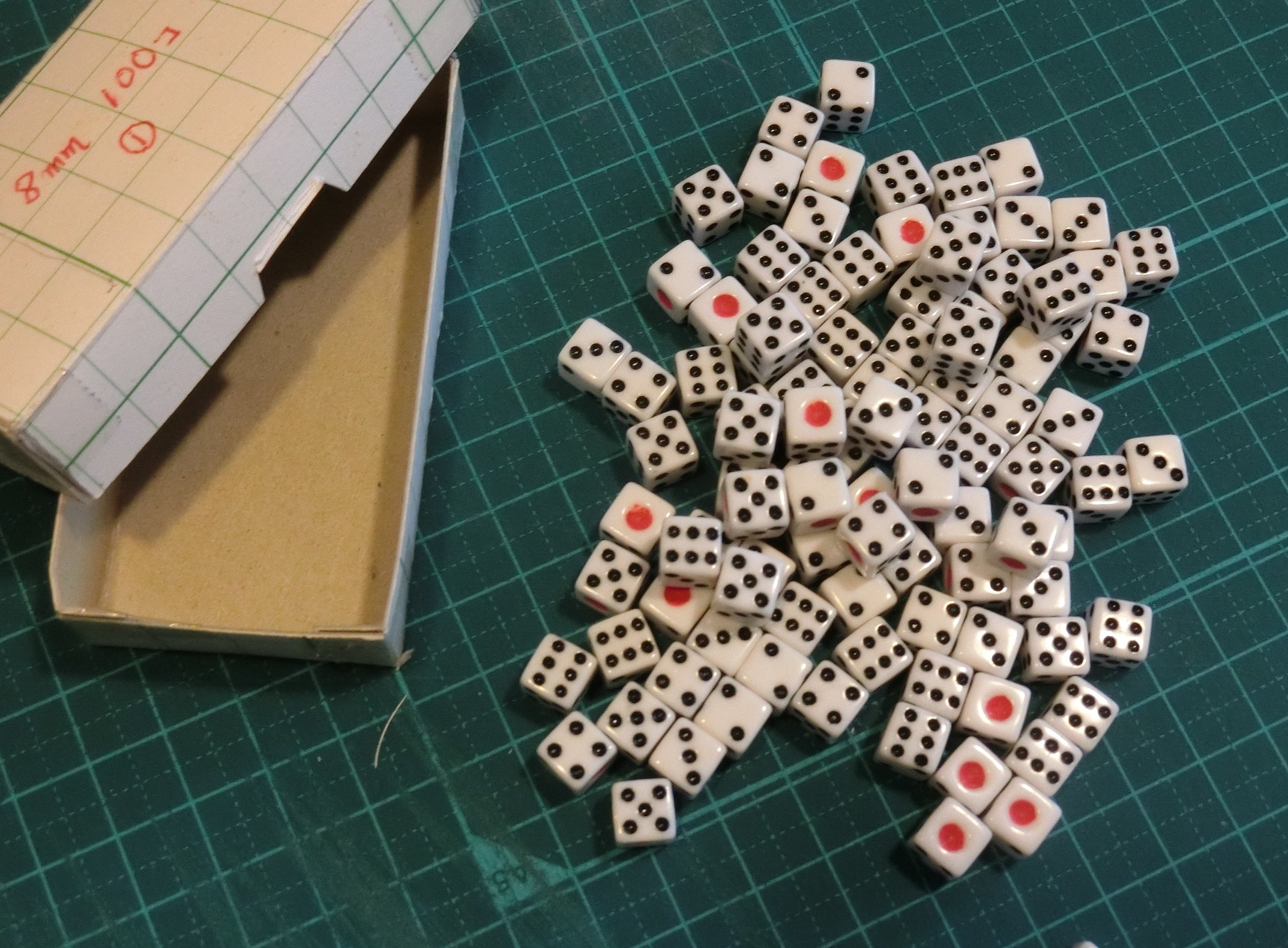I throw 100 dice and consider the number of eyes of ① which appeared to be the atom which collapsed. I remove the dice of ① for each once and count the number of left dice. It becomes the graph expressing an exponential function when I make the number of left dice a graph. It is unexpectedly pleasant to actually check the mathematics of the gamble. It is an experiment to swell plenty because ① does not readily appear in last one.

``````# Trial experiment of 100 dice by Python

import random as rnd
n = 100
while n > 0:           # It is repeated until n becomes 0
for i in range(n):
num = rnd.randint(1,6)     # To shoot dice
if num == 1:
n -= 1   #　If it is 1, remove it
print(n)``````

## Trial experiment to throw 100 dice, and to remove 1 eye

[ ]in a roll of the dice